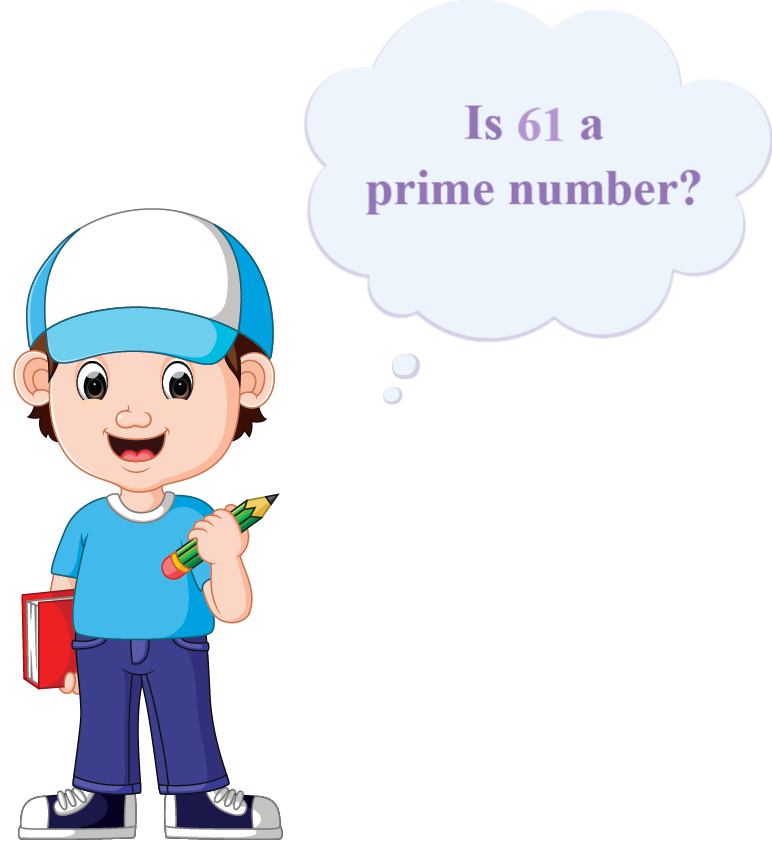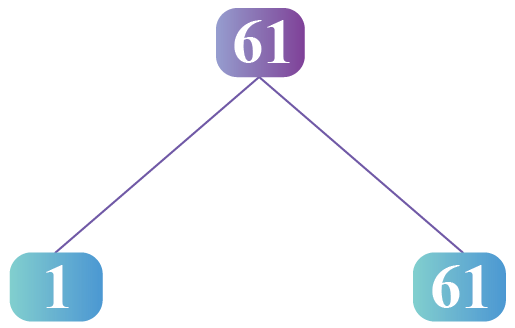# Is 61 a Prime Number

Is 61 a Prime Number
Go back to  'Prime Numbers'

Did you know that the first written evidence of prime numbers was found in 1550 BC?

If a number cannot be divided into equal groups, then it is known as a prime number.

Can you divide 61 into equal groups of 2? Equal groups of 3 ? Groups of 4?Let's find an answer to this question!

In this mini-lesson, we will explore the world of prime numbers by finding the answers to the questions like what is a prime number, is 61 a prime number, or if  61 is a composite number, etc., with the help of solved examples and interactive questions.

## Lesson Plan

 1 Is 61 a Prime Number? 2 Important Notes 3 Solved Examples 4 Thinking Out of the Box! 5 Interactive Questions

## What Is a Prime Number?

A natural number that is greater than 1 which cannot be expressed as a product of any two other numbers is a prime number.

For example, 91 has just two factors: 1 and 91

So, 91 is a prime number.

## Is 61 a Prime Number?

Let's determine the factors of 61 by prime factorization.So, 61 can be expressed as $$61=1\times61$$.

Clearly, it has only two factors.

So, 61 is a prime number.

## Is 61 a Composite Number?

composite number is a number that has more than two factors.

Moreover, if a number is not a prime number, then it is a composite number.

And vice versa is also true.

Therefore, 61 is not a composite number.

## Solved Examples

 Example 1

In a class, the teacher told four students to pick random prime numbers.

Tiron, Paul, Yasmin, and Lebron have chosen 49, 61, 63, and 91 respectively.

Who do you think has chosen a prime number?

Solution

We have 4 numbers: 49, 61, 63, and 91.

The number 49 is divisible by 7. So, it is not a prime number.

The number 63 is divisible by 7 and 9. So, it is not a prime number.

The number 91 is divisible by 7. So, it is not a prime number.

 So, the number chosen by Paul is a prime number.
 Example 2

Karen has to prove that 1 added or subtracted 61, makes it a composite number. How will she prove it?

Solution

On adding 1 to 61, Karen will get 62.

Factors of 62 =1, 2, 31, and 62.

Clearly, 62 has more than 2 factors. Thus, it is not a prime number.

On subtraction of 1 from 61, she will get 60.

Factors of 60: 1, 2, 3, 4, 5, 6, 10, 12, 15, 20, 30, and 60.

It has factors other than 1 and 60, which makes it a composite number.

Hence 1 added or subtracted in 61, makes it a composite number.

 $$\therefore$$1 added or subtracted in 61, makes it a composite number.Think Tank
1. What are the twin prime numbers?

## Interactive Questions

Here are a few activities for you to practice.

## Let's Summarize

The mini-lesson targeted the fascinating concept of knowing if 61 is a prime number or not. The math journey around the 61 being a prime number starts with what a student already knows, and goes on to creatively crafting a fresh concept in the young minds. Done in a way that not only it is relatable and easy to grasp, but also will stay with them forever. Here lies the magic with Cuemath.

At Cuemath, our team of math experts is dedicated to making learning fun for our favorite readers, the students!

Through an interactive and engaging learning-teaching-learning approach, the teachers explore all angles of a topic.

Be it worksheets, online classes, doubt sessions, or any other form of relation, it’s the logical thinking and smart learning approach that we, at Cuemath, believe in.

## 1. Why is 61 a prime number?

A natural number that is greater than 1 which cannot be expressed as a product of any two other numbers is a prime number.

Since 61 has just two factors: 1 and 61

Hence, 61 is a prime number.

## 2. What can 61 be divided by?

Divisors of 61 are the same as their factors i.e. 1, 3, 7, 9, 21, and 61.

## 3. Is 61 a prime number?

Yes, 61 is a prime number.

More Important Topics
Numbers
Algebra
Geometry
Measurement
Money
Data
Trigonometry
Calculus